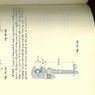# Statics: pipe wrench gripping pipe

• SoylentBlue
In summary, the book's answer key and the diagram show that the wrench has to grip the pipe and therefore we must have some Cy. Mathematically, I don't see how the 500N force produces any corresponding vertical reactions. If D is the fulcrum, you wind up with a Cx of 5000N and Cy of zero.f

## Homework Statement

A 500N force is applied to the handle at point F. The geometry & measurements are as given in the illustration. Find Cx, Cy, Ax and Ay[/B]

## Homework Equations

Well, the 500N force produces a 200Nm torque at point D, since DF is 400mm. [/B]

## The Attempt at a Solution

So the book's answer key shows a Cx of 4900N and Cy of 19,800N.
Intuitively, I see that the wrench has to grip the pipe and therefore we must have some Cy.
Mathematically, I don't see how the 500N force produces any corresponding vertical reactions. If D is the fulcrum, you wind up with a Cx of 5000N and Cy of zero.
Or, wait: if you want that pipe to turn, shouldn't the fulcrum be dead center of the pipe? Still not seeing how that fulcrum would yield any value for Cy.
Can someone please point me in the right direction?
The Internet is my professor...I'm trying to wade through statics by myself.[/B]

#### Attachments

•prob6104A.jpeg
36.8 KB · Views: 844
The pin at D is offset from center by 20 mm. You must consider that.
The book answers appear correct: Cx is not 5000 and Cy is not zero.
I would first determine Cx using the entire wrench with pipe removed and replaced with Cx, Cy, Ax, and Ay forces, then sum moments = 0 to solve for Cx. Once you find Cx, then look at a FBD of the lower part of the wrench cut through the pin at D to solve for Cy by summing moments appropriately.

•Tallus Bryne
Or, wait: if you want that pipe to turn, shouldn't the fulcrum be dead center of the pipe? Still not seeing how that fulcrum would yield any value for Cy.

Doesn't matter what kind of turning you envision for the pipe. A condition for static equilibrium is the sum of moments about any arbitrary point is zero. If you try to sum all moments of forces with respect to points which are on the line of action of Cy, you will never be able to solve for Cy. Like PhanthomJay was emphasizing in the beginning of his response, the offset of point D is critical in solving this problem.

Oh, I see.
I can't believe I missed something so basic!
OK, let's try again.
From D to C, we have a 20mm horizontal hop and a 40mm vertical hop, forming a triangle with a 63.4-degree angle. The hypotenuse is 44.72 mm.
So sum of moments about D is (.4m)(500N)-(.04472m)Fc.
That yields Fc, at a 26.6-degree angle off horizontal, of 4474N.
The horizontal component is (cos 26.6)Fc or 4014N; the vertical component is (sin26.6)Fc or 2000N.
Am I on the right track?
Thank you again for your input :^)

#### Attachments

No, you are assuming that Fc is perpendicular to the hypotenuse, which is not correct. See post 2 for where to start, summing moments about A to solve for Cx. You won't be able to find Cy yet. Once you solve for Cx, then you can proceed per your last attempt to sum moments about D to solve for Cy.

Thank you thank you thank you.
I spent more time than I will admit on this problem, but now I have conquered it!

OK, take the entire wrench as a single entity, and forget about the pipe.
Sum Fx: 500 + Cx + Ax=0
Sum Fy: Ay + Cy = 0
Sum M about A: (.05m)Cx + (.490m)(500N) = 0
So Cx = 4900N (agrees with book)
Ax = -4400 N (agrees with book)

We're halfway there!

Now look at FDC.
Sum Fx: 500 +Dx + Cx = 0
Sum Fy: Cy + Dy = 0
Sum M about D: -(.4m)(500N) + (.02m)Cy+ -(.04m)Cx = 0
Since we know Cx
Cy = 19,800 (agrees with book)

•Tallus Bryne
Good! Your plus and minus signs for moments about D are correct...I assume you put some thought into the proper directions of the forces and moments.